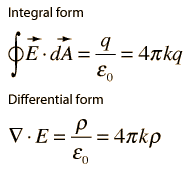Proof using Gauss' Law

Homework Statement

A long, thin, straight wire of length L has a positive charge Q distributed uniformly along it. Use Gauss' Law to show that the electric field created by this wire at a radial distance r has a magnitude of E = λ/(2πεor), where λ = Q/L. (Hint: For a Gaussian surface, use a cylinder aligned with its axis along the wire and now that the cylinder has a flat surface at either end, as well as a curved surface.

Homework EquationsThe Attempt at a Solution

I have no idea where to start! Please give me a push.

Attachments

When using Gauss' Law to derive the electric field from a known charge distribution, the Integral Form is your best friend.

Take their hint and imagine a surface that is a cylinder or radius r aligned along the axis of the the wire (that is to say, the axis of the cylinder coincides with the wire). Now, because of the cylindrical symmetry of the problem, we can be confident that the electric field will always point perpendicularly out from the wire (as long as we stay away from the ends of the wire where we get some fringe effects). To see this, imagine being an charge sitting somewhere above the wire. As long as the ends are really far away, there are just as many charges to your left (that try to push you to the right) as there are to your right (which try to push you to your left). So the left-right forces cancel out and you get pushed straight away from the wire. Also, you you feel the same push no matter where you were on your Gaussian surface (the cylinder) because you would be the same distance away from the wire, which is all that matters since the left-right forces cancel out.

Now that you know this, you need to think about the direction of the dA vector for different parts of your cylinder. On the body of the cylinder, it points in the same direction as the E-field: away from the wire. And on the caps it points perpendicularly to the wire. This should help you simply that the surface integral.

Can you take it from here?

A = 2(pi)rL

E[2(pi)rL] = Q/ε
E = Q/ε[2(pi)rL]
E = λ/2(pi)εr

Is this correct?

Bingo! And nice link LowlyPion; those illustrations are quite handy.

Q = λL

A = 2(pi)r

E = Q/Aε

Therefore:

E = λL/2(pi)rε

Correct me if I'm wrong because I need to know the answer too.